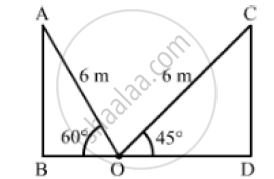# A Ladder of Length 6meters Makes an Angle of 45° with the Floor While Leaning Against One Wall of a Room. Find the Distance Between Two Walls of the Room. - Mathematics

A ladder of length 6meters makes an angle of 45° with the floor while leaning against one wall of a room. If the fort of the ladder is kept fixed on the floor and it is made to lean against the opposite wall of the room, it makes an angle of 60° with the floor. Find the
distance between two walls of the room.

#### SolutionLet AB and CD be the two opposite walls of the room and the foot of the ladder be fixed at
the point O on the ground.
We have,
AO = CO = 6m,∠AOB = 60° and ∠COD = 45°
In ΔABO,

cos 60°= (BD)/(AO)

⇒1/2 = (BO)/6

⇒ BO = 6/2

⇒ BO = 3m

Also, in ΔCDO,

cos 45° = (DO)/(CO)

⇒1/sqrt(2) = (DO)/6

⇒ DO = 6/sqrt(2) xx sqrt(2)/sqrt(2)

⇒DO = (6 sqrt(2))/2

⇒ Do = 3 sqrt(2) m

Now, the distance between two walls of the room = BD

=BO+DO

=3+3sqrt(2)

=3(1+sqrt(2))

=3(1+1.414)

=3(2.414)

=7.242

~~7.24m

So, the distant between two walls of the room is 7. 24 m.

Is there an error in this question or solution?
Chapter 14: Height and Distance - Exercises

#### APPEARS IN

RS Aggarwal Secondary School Class 10 Maths
Chapter 14 Height and Distance
Exercises | Q 30

Share